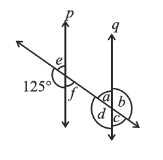Q

# In the adjoining figure, p is parallel to q. Find the unknown angles.

3. In the adjoining figure,. Find the unknown angles.Views

The angles can be found using different properties:

(a)         (The angles are linear pair)

(b)                              (Vertically opposite angle)

(c)                                            (Corresponding angle)

(d)                             (Vertically opposite angle)

(e)              (Vertically opposite angel,  linear pair).

Exams
Articles
Questions